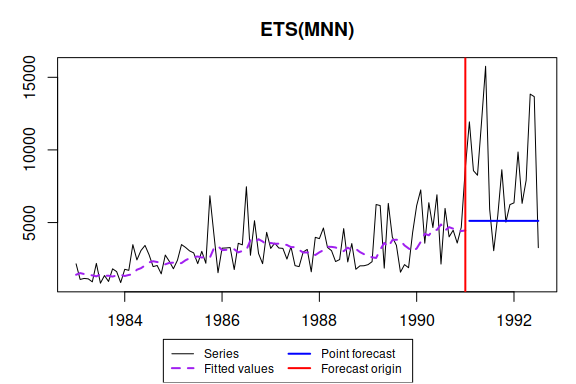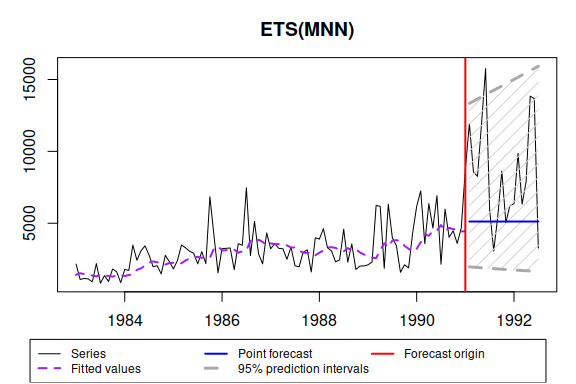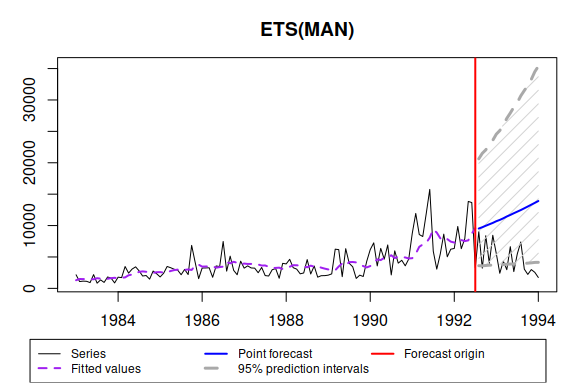# es() - Exponential Smoothing

#### 2021-04-15

es() is a part of smooth package. It allows constructing Exponential Smoothing (also known as ETS), selecting the most appropriate one among 30 possible ones, including exogenous variables and many more.

In this vignette we will use data from Mcomp package, so it is advised to install it. We also use some of the functions of the greybox package.

require(Mcomp)
require(smooth)
require(greybox)

You may note that Mcomp depends on forecast package and if you load both forecast and smooth, then you will have a message that forecast() function is masked from the environment. There is nothing to be worried about - smooth uses this function for consistency purposes and has exactly the same original forecast() as in the forecast package. The inclusion of this function in smooth was done only in order not to include forecast in dependencies of the package.

The simplest call of this function is:

es(M3$N2457$x, h=18, holdout=TRUE, silent=FALSE)
## Forming the pool of models based on... ANN, ANA, AAN, Estimation progress:    100%... Done!
## Time elapsed: 0.23 seconds
## Model estimated: ETS(MNN)
## Persistence vector g:
## alpha
## 0.154
## Initial values were optimised.
##
## Loss function type: likelihood; Loss function value: 834.9766
## Error standard deviation: 0.4889
## Sample size: 97
## Number of estimated parameters: 3
## Number of degrees of freedom: 94
## Information criteria:
##      AIC     AICc      BIC     BICc
## 1675.953 1676.211 1683.677 1684.268
##
## Forecast errors:
## MPE: 25.6%; sCE: 1892.7%; Bias: 86.3%; MAPE: 39.5%
## MASE: 2.92; sMAE: 119.1%; sMSE: 239.6%; rMAE: 1.247; rRMSE: 1.358In this case function uses branch and bound algorithm to form a pool of models to check and after that constructs a model with the lowest information criterion. As we can see, it also produces an output with brief information about the model, which contains:

1. How much time was elapsed for the model construction;
2. What type of ETS was selected;
3. Values of persistence vector (smoothing parameters);
4. What type of initialisation was used;
5. How many parameters were estimated (standard deviation is included);
6. Standard deviation of residuals. The model has multiplicative error term, so as a result the standard deviation is small.
7. Cost function type and the value of that cost function;
8. Information criteria for this model;
9. Forecast errors (because we have set holdout=TRUE).

The function has also produced a graph with actual values, fitted values and point forecasts.

If we need prediction interval, then we run:

es(M3$N2457$x, h=18, holdout=TRUE, interval=TRUE, silent=FALSE)
## Forming the pool of models based on... ANN, ANA, AAN, Estimation progress:    100%... Done!
## Time elapsed: 0.23 seconds
## Model estimated: ETS(MNN)
## Persistence vector g:
## alpha
## 0.154
## Initial values were optimised.
##
## Loss function type: likelihood; Loss function value: 834.9766
## Error standard deviation: 0.4889
## Sample size: 97
## Number of estimated parameters: 3
## Number of degrees of freedom: 94
## Information criteria:
##      AIC     AICc      BIC     BICc
## 1675.953 1676.211 1683.677 1684.268
##
## 95% parametric prediction interval was constructed
## 94% of values are in the prediction interval
## Forecast errors:
## MPE: 25.6%; sCE: 1892.7%; Bias: 86.3%; MAPE: 39.5%
## MASE: 2.92; sMAE: 119.1%; sMSE: 239.6%; rMAE: 1.247; rRMSE: 1.358Due to multiplicative nature of error term in the model, the interval are asymmetric. This is the expected behaviour. The other thing to note is that the output now also provides the theoretical width of prediction interval and its actual coverage.

If we save the model (and let’s say we want it to work silently):

ourModel <- es(M3$N2457$x, h=18, holdout=TRUE, silent="all")

we can then reuse it for different purposes:

es(M3$N2457$x, model=ourModel, h=18, holdout=FALSE, interval="np", level=0.93)
## Time elapsed: 0.02 seconds
## Model estimated: ETS(MNN)
## Persistence vector g:
## alpha
## 0.154
## Initial values were provided by user.
##
## Loss function type: likelihood; Loss function value: 1011.8864
## Error standard deviation: 0.5027
## Sample size: 115
## Number of estimated parameters: 3
## Number of provided parameters: 2
## Number of degrees of freedom: 112
## Information criteria:
##      AIC     AICc      BIC     BICc
## 2025.773 2025.808 2028.518 2028.602
##
## 93% nonparametric prediction interval was constructed

We can also extract the type of model in order to reuse it later:

modelType(ourModel)
##  "MNN"

This handy function, by the way, also works with ets() from forecast package.

If we need actual values from the model, we can use actuals() method from greybox package:

actuals(ourModel)
##         Jan    Feb    Mar    Apr    May    Jun    Jul    Aug    Sep    Oct
## 1983 2158.1 1086.4 1154.7 1125.6  920.0 2188.6  829.2 1353.1  947.2 1816.8
## 1984 1783.3 1713.1 3479.7 2429.4 3074.3 3427.4 2783.7 1968.7 2045.6 1471.3
## 1985 1821.0 2409.8 3485.8 3289.2 3048.3 2914.1 2173.9 3018.4 2200.1 6844.3
## 1986 3238.9 3252.2 3278.8 1766.8 3572.8 3467.6 7464.7 2748.4 5126.7 2870.8
## 1987 3220.7 3586.0 3249.5 3222.5 2488.5 3332.4 2036.1 1968.2 2967.2 3151.6
## 1988 3894.1 4625.5 3291.7 3065.6 2316.5 2453.4 4582.8 2291.2 3555.5 1785.0
## 1989 2102.9 2307.7 6242.1 6170.5 1863.5 6318.9 3992.8 3435.1 1585.8 2106.8
## 1990 6168.0 7247.4 3579.7 6365.2 4658.9 6911.8 2143.7 5973.9 4017.2 4473.0
## 1991 8749.1
##         Nov    Dec
## 1983 1624.5  868.5
## 1984 2763.7 2328.4
## 1985 4160.4 1548.8
## 1986 2170.2 4326.8
## 1987 1610.5 3985.0
## 1988 2020.0 2026.8
## 1989 1892.1 4310.6
## 1990 3591.9 4676.5
## 1991

We can then use persistence or initials only from the model to construct the other one:

es(M3$N2457$x, model=modelType(ourModel), h=18, holdout=FALSE, initial=ourModel$initial, silent="graph") ## Time elapsed: 0.01 seconds ## Model estimated: ETS(MNN) ## Persistence vector g: ## alpha ## 0.2219 ## Initial values were provided by user. ## ## Loss function type: likelihood; Loss function value: 1011.2259 ## Error standard deviation: 0.491 ## Sample size: 115 ## Number of estimated parameters: 2 ## Number of provided parameters: 1 ## Number of degrees of freedom: 113 ## Information criteria: ## AIC AICc BIC BICc ## 2026.452 2026.559 2031.942 2032.196 es(M3$N2457$x, model=modelType(ourModel), h=18, holdout=FALSE, persistence=ourModel$persistence, silent="graph")
## Time elapsed: 0.01 seconds
## Model estimated: ETS(MNN)
## Persistence vector g:
## alpha
## 0.154
## Initial values were optimised.
##
## Loss function type: likelihood; Loss function value: 1011.8809
## Error standard deviation: 0.5055
## Sample size: 115
## Number of estimated parameters: 2
## Number of provided parameters: 1
## Number of degrees of freedom: 113
## Information criteria:
##      AIC     AICc      BIC     BICc
## 2027.762 2027.869 2033.252 2033.506

or provide some arbitrary values:

es(M3$N2457$x, model=modelType(ourModel), h=18, holdout=FALSE, initial=1500, silent="graph")
## Time elapsed: 0.01 seconds
## Model estimated: ETS(MNN)
## Persistence vector g:
##  alpha
## 0.2248
## Initial values were provided by user.
##
## Loss function type: likelihood; Loss function value: 1011.2742
## Error standard deviation: 0.4897
## Sample size: 115
## Number of estimated parameters: 2
## Number of provided parameters: 1
## Number of degrees of freedom: 113
## Information criteria:
##      AIC     AICc      BIC     BICc
## 2026.548 2026.656 2032.038 2032.292

Using some other parameters may lead to completely different model and forecasts:

es(M3$N2457$x, h=18, holdout=TRUE, loss="aTMSE", bounds="a", ic="BIC", interval=TRUE)
## Time elapsed: 0.21 seconds
## Model estimated: ETS(ANN)
## Persistence vector g:
##  alpha
## 0.0798
## Initial values were optimised.
##
## Loss function type: aTMSE; Loss function value: 39565651.9
## Error standard deviation: 1466.912
## Sample size: 97
## Number of estimated parameters: 3
## Number of degrees of freedom: 94
## Information criteria:
##      AIC     AICc      BIC     BICc
## 1972.365 1974.736 1985.865 1986.455
##
## 95% parametric prediction interval was constructed
## 44% of values are in the prediction interval
## Forecast errors:
## MPE: 33.4%; sCE: 2196.8%; Bias: 90.4%; MAPE: 43.4%
## MASE: 3.235; sMAE: 132%; sMSE: 278%; rMAE: 1.382; rRMSE: 1.463

You can play around with all the available parameters to see what’s their effect on final model.

In order to combine forecasts we need to use “C” letter:

es(M3$N2457$x, model="CCN", h=18, holdout=TRUE, silent="graph")
## Estimation progress:    10%20%30%40%50%60%70%80%90%100%... Done!
## Time elapsed: 0.4 seconds
## Model estimated: ETS(CCN)
## Initial values were optimised.
##
## Loss function type: likelihood
## Error standard deviation: 1353.486
## Sample size: 97
## Information criteria:
## (combined values)
##     AIC    AICc     BIC    BICc
## 93.0808 93.1026 93.5988 93.6450
##
## Forecast errors:
## MPE: 19.2%; sCE: 1652.4%; Bias: 80.7%; MAPE: 37.9%
## MASE: 2.727; sMAE: 111.3%; sMSE: 216%; rMAE: 1.165; rRMSE: 1.289

Model selection from a specified pool and forecasts combination are called using respectively:

es(M3$N2457$x, model=c("ANN","AAN","AAdN","ANA","AAA","AAdA"), h=18, holdout=TRUE, silent="graph")
## Estimation progress:    17%33%50%67%83%100%... Done!
## Time elapsed: 0.42 seconds
## Model estimated: ETS(ANN)
## Persistence vector g:
##  alpha
## 0.1582
## Initial values were optimised.
##
## Loss function type: likelihood; Loss function value: 841.4934
## Error standard deviation: 1439.368
## Sample size: 97
## Number of estimated parameters: 3
## Number of degrees of freedom: 94
## Information criteria:
##      AIC     AICc      BIC     BICc
## 1688.987 1689.245 1696.711 1697.301
##
## Forecast errors:
## MPE: 25.3%; sCE: 1880.4%; Bias: 86%; MAPE: 39.4%
## MASE: 2.909; sMAE: 118.7%; sMSE: 238.1%; rMAE: 1.243; rRMSE: 1.354
es(M3$N2457$x, model=c("CCC","ANN","AAN","AAdN","ANA","AAA","AAdA"), h=18, holdout=TRUE, silent="graph")
## Estimation progress:    17%33%50%67%83%100%... Done!
## Time elapsed: 0.42 seconds
## Model estimated: ETS(CCC)
## Initial values were optimised.
##
## Loss function type: likelihood
## Error standard deviation: 1324.289
## Sample size: 97
## Information criteria:
## (combined values)
##     AIC    AICc     BIC    BICc
## 94.1842 94.3313 95.1020 95.1421
##
## Forecast errors:
## MPE: 9.3%; sCE: 1253%; Bias: 61.9%; MAPE: 35.7%
## MASE: 2.428; sMAE: 99.1%; sMSE: 177.3%; rMAE: 1.037; rRMSE: 1.168

Now let’s introduce some artificial exogenous variables:

x <- cbind(rnorm(length(M3$N2457$x),50,3),rnorm(length(M3$N2457$x),100,7))

and fit a model with all the exogenous first:

es(M3$N2457$x, model="ZZZ", h=18, holdout=TRUE, xreg=x)
## Time elapsed: 0.4 seconds
## Model estimated: ETSX(MNN)
## Persistence vector g:
##  alpha
## 0.1428
## Initial values were optimised.
## Xreg coefficients were estimated in a normal style
##
## Loss function type: likelihood; Loss function value: 829.391
## Error standard deviation: 0.4715
## Sample size: 97
## Number of estimated parameters: 7
## Number of degrees of freedom: 90
## Information criteria:
##      AIC     AICc      BIC     BICc
## 1668.782 1669.441 1681.656 1683.164
##
## Forecast errors:
## MPE: 26.3%; sCE: 1874.1%; Bias: 87.2%; MAPE: 37.7%
## MASE: 2.84; sMAE: 115.8%; sMSE: 230.7%; rMAE: 1.213; rRMSE: 1.333

or construct a model with selected exogenous (based on IC):

es(M3$N2457$x, model="ZZZ", h=18, holdout=TRUE, xreg=x, xregDo="select")
## Time elapsed: 1 seconds
## Model estimated: ETSX(MNN)
## Persistence vector g:
##  alpha
## 0.1428
## Initial values were optimised.
## Xreg coefficients were estimated in a normal style
##
## Loss function type: likelihood; Loss function value: 829.391
## Error standard deviation: 0.4715
## Sample size: 97
## Number of estimated parameters: 7
## Number of degrees of freedom: 90
## Information criteria:
##      AIC     AICc      BIC     BICc
## 1668.782 1669.441 1681.656 1683.164
##
## Forecast errors:
## MPE: 26.3%; sCE: 1874.1%; Bias: 87.2%; MAPE: 37.7%
## MASE: 2.84; sMAE: 115.8%; sMSE: 230.7%; rMAE: 1.213; rRMSE: 1.333

If we want to check if lagged x can be used for forecasting purposes, we can use xregExpander() function from greybox package:

es(M3$N2457$x, model="ZZZ", h=18, holdout=TRUE, xreg=xregExpander(x), xregDo="select")
## Time elapsed: 1.07 seconds
## Model estimated: ETSX(MNN)
## Persistence vector g:
##  alpha
## 0.1428
## Initial values were optimised.
## Xreg coefficients were estimated in a normal style
##
## Loss function type: likelihood; Loss function value: 829.391
## Error standard deviation: 0.4715
## Sample size: 97
## Number of estimated parameters: 7
## Number of degrees of freedom: 90
## Information criteria:
##      AIC     AICc      BIC     BICc
## 1668.782 1669.441 1681.656 1683.164
##
## Forecast errors:
## MPE: 26.3%; sCE: 1874.1%; Bias: 87.2%; MAPE: 37.7%
## MASE: 2.84; sMAE: 115.8%; sMSE: 230.7%; rMAE: 1.213; rRMSE: 1.333

If we are confused about the type of estimated model, the function formula() will help us:

formula(ourModel)
##  "y[t] = l[t-1] * e[t]"

Finally, if you work with M or M3 data, and need to test a function on a specific time series, you can use the following simplified call:

es(M3\$N2457, interval=TRUE, silent=FALSE)
## Forming the pool of models based on... ANN, ANA, AAN, Estimation progress:    40%50%60%70%80%90%100%... Done!
## Time elapsed: 1.76 seconds
## Model estimated: ETS(MAN)
## Persistence vector g:
##  alpha   beta
## 0.1641 0.0000
## Initial values were optimised.
##
## Loss function type: likelihood; Loss function value: 1008.21
## Error standard deviation: 0.4461
## Sample size: 115
## Number of estimated parameters: 4
## Number of provided parameters: 1
## Number of degrees of freedom: 111
## Information criteria:
##      AIC     AICc      BIC     BICc
## 2024.420 2024.784 2035.400 2036.262
##
## 95% parametric prediction interval was constructed
## 50% of values are in the prediction interval
## Forecast errors:
## MPE: -232.9%; sCE: -3154%; Bias: -100%; MAPE: 232.9%
## MASE: 4.272; sMAE: 175.2%; sMSE: 374.9%; rMAE: 3.554; rRMSE: 2.859This command has taken the data, split it into in-sample and holdout and produced the forecast of appropriate length to the holdout.